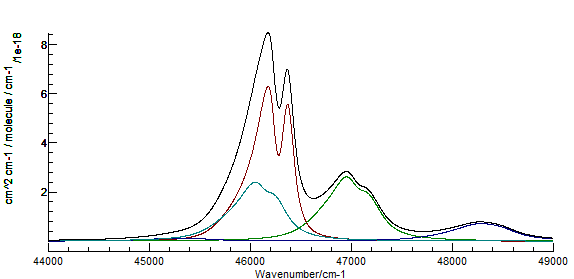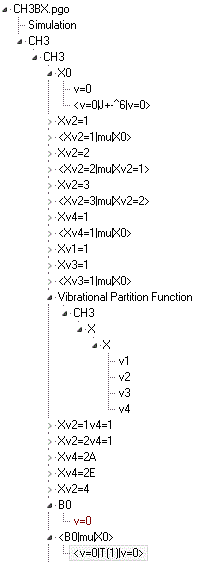Molecule Types Symmetric Tops Samples

# The B̃2A1'−X̃2A2″ transition in CH3

The files here are from "Reanalysis of Rate Data for the Reaction CH3 + CH3 → C2H6 Using Revised Cross Sections and a Linearized Second-Order Master Equation", M. A. Blitz, N. J. B. Green, R. J. Shannon, M. J. Pilling, P. W. Seakins, C. M. Western, and S. H. Robertson, J. Phys. Chem. A, (2015), doi:10.1021/acs.jpca.5b01002.CH3BX.pgo is the file used to produce the left side figure 1 of the paper, with some minor modifications. It is a relatively complicated example, containing multiple vibrational levels in both the B and X states, and has been produced, as discussed in the paper, by considering as much of the available literature as possible. Notes:
• A Vibrational Partition Function object is used to allow a complete partition function to be calculated. This adds a Vibrating Molecule object to calculate the vibrational partition function under the Symmetric Molecule object.For this to work correctly, all the vibrational levels below ESwitch (here 3200 cm-1) need to be explicitly included in the calculation, which in this case required a few states (such as 4ν2) to be included with estimated constants. The partition function is then calculated by an explicit sum over levels, including rotational levels for vibrational levels below 3200 cm-1, and a sum over vibrational levels above this, assuming a constant ratio between the rotational and vibrational parts.
• The B state levels all include rotational levels widths due to predissociation, set by using Width, wK and wJ.
• The X state rotational constants were obtained by a fit to the available observed transitions. The fit files used are included as an example. CH3.obs is the (small) main file, which simply includes one file for each literature source: CanJPhys_79_449.obs, JCP_75_5256.obs and JCP_96_1822.obs.
• The B-X intensities are discussed in detail in the paper; note that the correction factor of 1.2 mentioned in the paper has bee included in the transition moments (they have been multiplied by 1.2½) so no additional correction should be necessary.
• Transition moments for selected infra-red vibrational transitions within the ground state are included in the file. These are based on literature values, but have not been subject to the same validation as the energy levels.
For completeness, the file(CH3simplevibBX.pgo) used to produce the vibrational background are also included. Given the approximate nature of the simulation, these are less likely to be of general use. An implementation restriction required the use of a linear point group rather than D3h. The .pgo file includes an example of a Table used with a Custom Transition Moment Function to remove selected transitions from the simulation. The observation file CH3simplevib.obs was used to determine some of the vibrational constants; to use this the table must be set inactive.